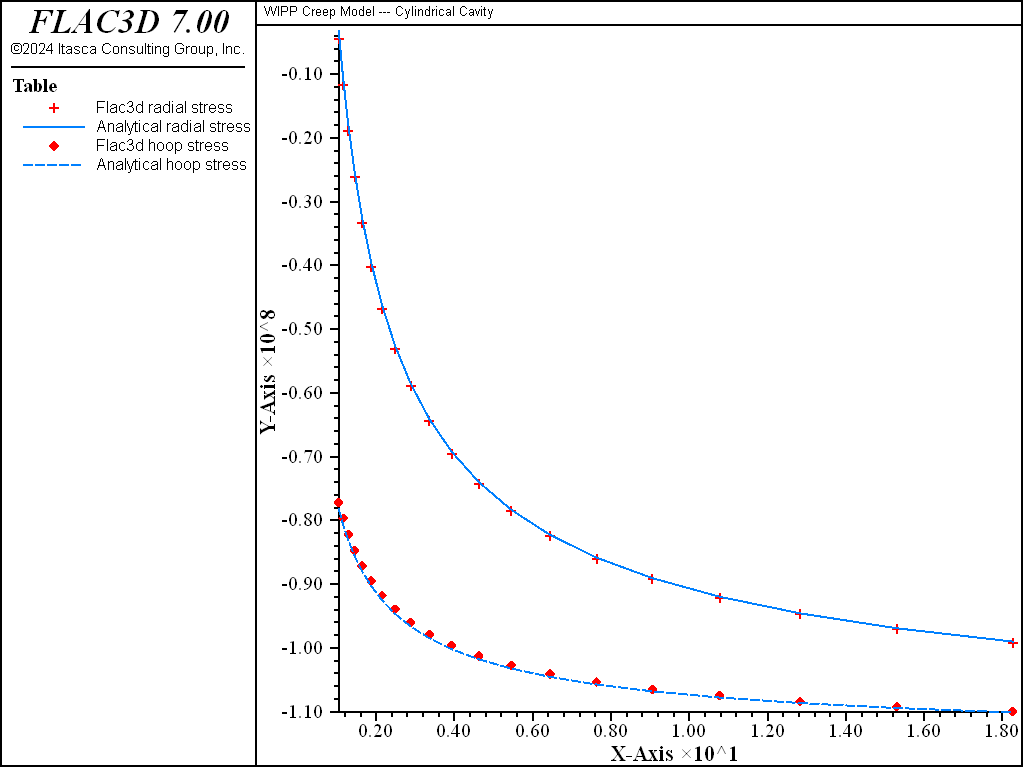# WIPP Model: Cylindrical Cavity

Note

To view this project in FLAC3D, use the menu command Help ► Examples…. Choose “Creep/CylindricalCavityWIPP” and select “CylindricalCavityWIPP.prj” to load. The project’s main data files are shown at the end of this example.

The WIPP-reference creep model in FLAC3D is used to solve the problem of radial creep of an infinitely long, thick-walled cylinder subjected to a pressure on its outer surface. The analytical steady-state solution, assuming that creep is defined by a single-component power law, is provided in Power Model: Cylindrical Cavity. The WIPP model can be converted to a power law formulation by using only the secondary creep-strain component. This is achieved by setting the WIPP properties a_wipp and b_wipp to zero. The WIPP model is then reduced to the form

$\dot \epsilon_{cr} = A \bar \sigma^n$

where $$A = D \exp(-Q/RT)$$.

For this problem, $$Q$$ = 12,000 cal/mol, $$R$$ = 1.987 cal/mol K, $$T$$ = 300° K, $$D$$ = 5.5299 × 10-17 (or $$A$$ = 1 × 10-25 Pa-3 yr-1), and $$n$$ = 3. The elastic properties of the material are $$E$$ = 820 MPa and $$\nu$$ = 0.3636.

The model uses the same FLAC3D grid and the same boundary conditions as as those described in Power Model: Cylindrical Cavity.

The results of this example are summarized in Figure 1 and Figure 2. Figure 1 compares the analytical solution for the radial velocity in the steady-state condition with the FLAC3D results. Figure 2 shows the comparison of radial and hoop stresses. The agreement between results is similar to that for the power law test in Power Model: Cylindrical Cavity.Figure 1: Comparison of radial velocity at steady state—FLAC3D WIPP model (symbol), analytical (line) versus radial distance.Figure 2: Comparison of radial and hoop stress at steady state—FLAC3D WIPP model radial stress (symbol), analytical radial stress (line), FLAC3D WIPP model hoop stress (symbol), analytical hoop stress (line) versus radial distance.

Data File

;------------------------------------------------------------
;       cylindrical cavity -- WIPP model
;-------------------------------------------------------------------
model new
model large-strain off
fish automatic-create off
model title "WIPP Creep Model --- Cylindrical Cavity"
model configure creep
;
zone create brick  point 0 1 0 0               point 1 20 0 0           ...
point 3 0.98769 0.15643 0   point 6 19.754 3.129 0   ...
point 2 1 0 -1              point 4 20 0 -1          ...
point 5 0.98769 0.15643 -1  point 7 19.754 3.129 -1  ...
size 20 1 1  ratio 1.2 1 1
zone face skin
zone cmodel wipp
; Properties and stresses in Pascal units (not MPa)
zone property bulk=1e9 shear=3e8
zone property constant-gas 1.987 activation-energy 12e3 exponent 3 ...
constant-d 5.5299e-17
zone property constant-a 0 constant-b 0 creep-rate-critical 5.39e-8 ...
temperature 300
;
zone gridpoint fix velocity-y range position-y 0
zone gridpoint fix velocity-z range position-y 0
zone face apply velocity-normal 0 range group 'North'
zone face apply velocity-dip    0 range group 'North'
zone face apply stress-normal -100e6 range group 'East'
zone initialize stress xx -100e6 yy -100e6 zz -100e6
;
model step 2000
model save 'cyl_wl'
;
model history mechanical unbalanced-maximum
zone history velocity-x gridpointid 1
zone history velocity-x gridpointid 81
zone history displacement-x gridpointid 1
zone history displacement-x gridpointid 81
zone history stress-xx gridpointid 1
zone history stress-yy gridpointid 1
zone history stress-zz gridpointid 1
model history creep time-total
model history timestep
;
zone gridpoint initialize velocity (0,0,0)
model creep active on
model creep timestep starting 1.0e-3
model creep timestep minimum 1.0e-4
model creep timestep maximum 1.0
model solve time-total 2000
model save 'cyl_w2'
; compare analytic solution......
program call 'solution' suppress
table  '1' label 'Flac3d x-velocity'
table '11' label 'Analytical x-velocity'
table  '2' label 'Flac3d radial stress'
table '12' label 'Analytical radial stress'
table  '3' label 'Flac3d hoop stress'
table '13' label 'Analytical hoop stress'
model save 'cylindrical-cavity-wipp'